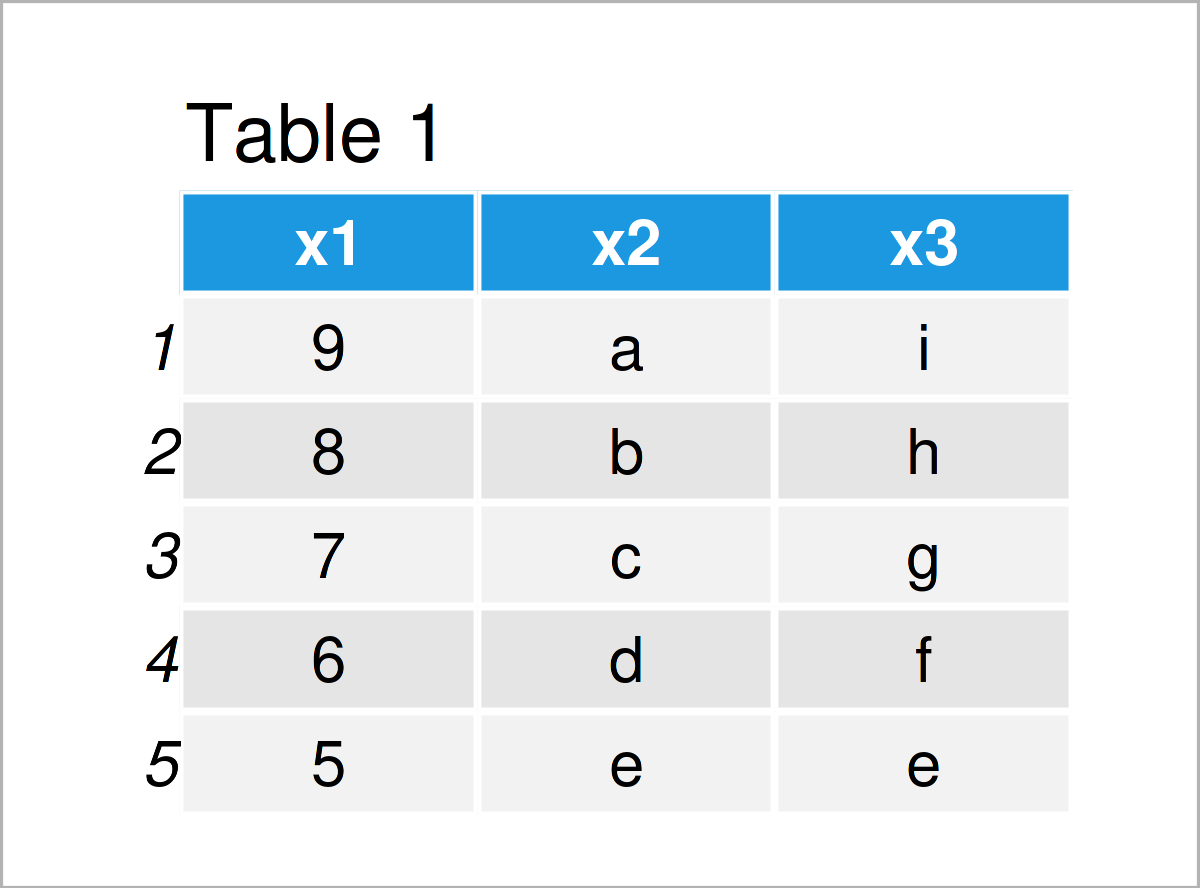# View() Function in R (Example)

In this article, I’ll explain how to apply the View() function to open a spreadsheet-style data viewer in R.

The page will contain one example for the application of the View() function. More precisely, the page looks as follows:

Let’s do this…

## Creation of Example Data

The first step is to create some exemplifying data:

```data <- data.frame(x1 = 9:5, # Create example data x2 = letters[1:5], x3 = letters[9:5]) data # Print example data```As you can see based on Table 1, our example data is a data frame consisting of five rows and three columns.

Let’s assume that we want to inspect this data frame within RStudio

## Example: Apply View() Function to Data Frame

This example explains how to use the View function to open a new window in RStudio, which illustrates the structure of a data frame.

For this, we have to apply the View command to our example data frame as shown below:

`View(data) # Apply View function`Table 2 illustrates how the new window in RStudio looks like. As you can see, this new window shows the rows and columns of our example data frame in a spreadsheet-style data viewer.

In this example, we have applied the Viewer function to a data frame object. However, please note that the Viewer function could also be applied to other data types such as matrices or tibbles, as long as these data sets can be coerced to a data frame with non-zero numbers of rows and columns.

## Video & Further Resources

I have recently published a video on my YouTube channel, which shows the R programming syntax of this tutorial. You can find the video below:

Furthermore, you could read the related articles on my homepage:

To summarize: In this tutorial you have learned how to apply the View() function to invoke a data viewer in the R programming language. Let me know in the comments section below, if you have any additional comments and/or questions.

Subscribe to the Statistics Globe Newsletter